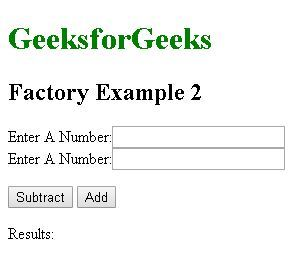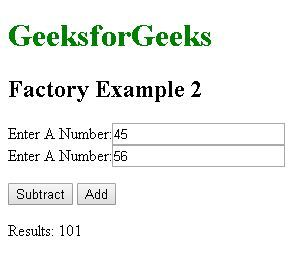Related Articles
AngularJS | Factory Method
• Last Updated : 30 Aug, 2019

AngularJS Factory Method makes the development process of AngularJS application more robust. A factory is a simple function which allows us to add some logic to a created object and return the created object. The factory is also used to create/return a function in the form of reusable code which can be used anywhere within the application. Whenever we create an object using factory it always returns a new instance for that object. The object returned by the factory can be integrated(injectible) with different components of the Angularjs framework such as controller, service, filter or directive.

Use:
In practical Scenario Factory generally acts as container or class for a collection of functions which fulfills different features of the application. When using with a constructor function it can be initiated within different Controllers.

Syntax:

`module.factory( 'factoryName', function(){ Custom code....});`

Example:
The Following example illustrate the use of factory code instantiated inside a controller to generate a random number

 ```<``html``>`` ` `<``head``>``    ``<``title``>Factory Example 1`` ` `    ``<``script` `src``=``"https://ajax.googleapis.com/ajax/libs/angularjs/1.6.9/angular.min.js"``>``    ````    ``<``script``>``        ``var application = angular.module('myApp', []);`` ` `        ``application.factory('random', function() {``            ``var randomObject = {};``            ``var number = Math.floor(Math.random() * 100);``            ``randomObject.generate = function() {``                ``return number;``            ``};``            ``return randomObject;``        ``});``       ` `        ``application.controller('thisapp', function(\$scope, random) {``            ``\$scope.generateRandom = function() {``                ``\$scope.randomNumber = random.generate();``            ``};``        ``});``    `````` ` `<``body``>``    ``<``h1` `style``=``"color:green"``>GeeksforGeeks``    ``<``h2``>Factory Examples``    ``<``div` `ng-app``=``"myApp"` `ng-controller``=``"thisapp"``>``        ``<``button` `ng-click``=``"generateRandom()"``>``          ``Generate Random Number``      ````        ``<``br``>{{randomNumber}}``    `````` ` ``

Output:On Clicking the generate random number button we get a different number every time. In this example, we use the factory method to define a function which carries a variable and using the Math.random we store a random value to that variable every time this function is called. This function is then called in the controller whose \$scope variable carries the random value from the called function we then call this controller to our HTML code to display the result.

Example: This example make use of factory to create a function to find addition or subtraction of two numbers. this function is then loaded in the controller \$scope variable which passes them to the html code for displaying the results.

 ```<``html``>`` ` `<``head``>``    ``<``title``>Factory Example 2``    ``<``script` `src``=``"https://ajax.googleapis.com/ajax/libs/angularjs/1.6.9/angular.min.js"``>``    ````    ``<``script``>``        ``var application = angular.module('myApp', []);`` ` `        ``application.factory('MyFactoryService', function() {``            ``var factory = {};``            ``factory.Subtract = function(a, b) {``                ``return a - b;``            ``};``            ``factory.Add = function(a, b) {``                ``return a + b;``            ``};``            ``return factory;``        ``});`` ` `        ``application.controller('thisapp', function(``                               ``\$scope, MyFactoryService) {``            ``\$scope.result = function() {``                ``\$scope.results = ``                  ``MyFactoryService.Subtract(\$scope.num1, \$scope.num2)``            ``};``            ``\$scope.result2 = function() {``                ``\$scope.results = ``                  ``MyFactoryService.Add(\$scope.num1, \$scope.num2)``            ``};``        ``});``    `````` ` `<``body``>``    ``<``h1` `style``=``"color:green"``>GeeksforGeeks``    ``<``h2``>Factory Example 2``    ``<``div` `ng-app``=``"myApp"` `ng-controller``=``"thisapp"``>``        ``<``p``>``            ``Enter A Number:``            ``<``input` `type``=``"number"` `ng-model``=``"num1"` `/>``            ``<``br``/> Enter A Number:``            ``<``input` `type``=``"number"` `ng-model``=``"num2"` `/>``            ``<``br``/>``        ````        ``<``button` `ng-click``=``"result()"``>Subtract``        ``<``button` `ng-click``=``"result2()"``>Add``        ``<``p``>Results: {{results}}``    `````` ` ``

Output:
Before:After entering the input clicking on the add/subtract button displays the result:My Personal Notes arrow_drop_up